Search by Topic

Resources tagged with Visualising similar to Squayles:

Filter by: Content type:
Age range:
Challenge level:

There are 180 results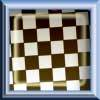Diagonal Dodge

Age 7 to 14 Challenge Level:

A game for 2 players. Can be played online. One player has 1 red counter, the other has 4 blue. The red counter needs to reach the other side, and the blue needs to trap the red.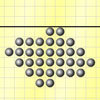Conway's Chequerboard Army

Age 11 to 14 Challenge Level:

Here is a solitaire type environment for you to experiment with. Which targets can you reach?Khun Phaen Escapes to Freedom

Age 11 to 14 Challenge Level:

Slide the pieces to move Khun Phaen past all the guards into the position on the right from which he can escape to freedom.Jam

Age 14 to 16 Challenge Level:

To avoid losing think of another very well known game where the patterns of play are similar.Cuboids

Age 11 to 14 Challenge Level:

Find a cuboid (with edges of integer values) that has a surface area of exactly 100 square units. Is there more than one? Can you find them all?Square It

Age 11 to 16 Challenge Level:

Players take it in turns to choose a dot on the grid. The winner is the first to have four dots that can be joined to form a square.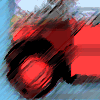Buses

Age 11 to 14 Challenge Level:

A bus route has a total duration of 40 minutes. Every 10 minutes, two buses set out, one from each end. How many buses will one bus meet on its way from one end to the other end?Triangles to Tetrahedra

Age 11 to 14 Challenge Level:

Imagine you have an unlimited number of four types of triangle. How many different tetrahedra can you make?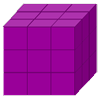Cubist Cuts

Age 11 to 14 Challenge Level:

A 3x3x3 cube may be reduced to unit cubes in six saw cuts. If after every cut you can rearrange the pieces before cutting straight through, can you do it in fewer?Coloured Edges

Age 11 to 14 Challenge Level:

The whole set of tiles is used to make a square. This has a green and blue border. There are no green or blue tiles anywhere in the square except on this border. How many tiles are there in the set?Triangle Inequality

Age 11 to 14 Challenge Level:

ABC is an equilateral triangle and P is a point in the interior of the triangle. We know that AP = 3cm and BP = 4cm. Prove that CP must be less than 10 cm.Bands and Bridges: Bringing Topology Back

Age 7 to 14

Lyndon Baker describes how the Mobius strip and Euler's law can introduce pupils to the idea of topology.Coordinate Patterns

Age 11 to 14 Challenge Level:

Charlie and Alison have been drawing patterns on coordinate grids. Can you picture where the patterns lead?Reflecting Squarely

Age 11 to 14 Challenge Level:

In how many ways can you fit all three pieces together to make shapes with line symmetry?Sliding Puzzle

Age 11 to 16 Challenge Level:

The aim of the game is to slide the green square from the top right hand corner to the bottom left hand corner in the least number of moves.Cubic Net

Age 14 to 18 Challenge Level:

This is an interactive net of a Rubik's cube. Twists of the 3D cube become mixes of the squares on the 2D net. Have a play and see how many scrambles you can undo!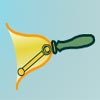You Owe Me Five Farthings, Say the Bells of St Martin's

Age 11 to 14 Challenge Level:

Use the interactivity to listen to the bells ringing a pattern. Now it's your turn! Play one of the bells yourself. How do you know when it is your turn to ring?Isosceles Triangles

Age 11 to 14 Challenge Level:

Draw some isosceles triangles with an area of $9$cm$^2$ and a vertex at (20,20). If all the vertices must have whole number coordinates, how many is it possible to draw?On the Edge

Age 11 to 14 Challenge Level:

If you move the tiles around, can you make squares with different coloured edges?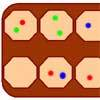Wari

Age 14 to 16 Challenge Level:

This is a simple version of an ancient game played all over the world. It is also called Mancala. What tactics will increase your chances of winning?Concrete Wheel

Age 11 to 14 Challenge Level:

A huge wheel is rolling past your window. What do you see?Jam

Age 14 to 16 Challenge Level:

A game for 2 playersZooming in on the Squares

Age 7 to 14

Start with a large square, join the midpoints of its sides, you'll see four right angled triangles. Remove these triangles, a second square is left. Repeat the operation. What happens?Sea Defences

Age 7 to 14 Challenge Level:

These are pictures of the sea defences at New Brighton. Can you work out what a basic shape might be in both images of the sea wall and work out a way they might fit together?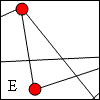Travelling Salesman

Age 11 to 14 Challenge Level:

A Hamiltonian circuit is a continuous path in a graph that passes through each of the vertices exactly once and returns to the start. How many Hamiltonian circuits can you find in these graphs?Tetrahedra Tester

Age 11 to 14 Challenge Level:

An irregular tetrahedron is composed of four different triangles. Can such a tetrahedron be constructed where the side lengths are 4, 5, 6, 7, 8 and 9 units of length?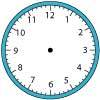Right Time

Age 11 to 14 Challenge Level:

At the time of writing the hour and minute hands of my clock are at right angles. How long will it be before they are at right angles again?Paving Paths

Age 11 to 14 Challenge Level:

How many different ways can I lay 10 paving slabs, each 2 foot by 1 foot, to make a path 2 foot wide and 10 foot long from my back door into my garden, without cutting any of the paving slabs?Picturing Triangular Numbers

Age 11 to 14 Challenge Level:

Triangular numbers can be represented by a triangular array of squares. What do you notice about the sum of identical triangle numbers?Inside Out

Age 14 to 16 Challenge Level:

There are 27 small cubes in a 3 x 3 x 3 cube, 54 faces being visible at any one time. Is it possible to reorganise these cubes so that by dipping the large cube into a pot of paint three times you. . . .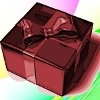Christmas Boxes

Age 11 to 14 Challenge Level:

Find all the ways to cut out a 'net' of six squares that can be folded into a cube.Convex Polygons

Age 11 to 14 Challenge Level:

Show that among the interior angles of a convex polygon there cannot be more than three acute angles.Framed

Age 11 to 14 Challenge Level:

Seven small rectangular pictures have one inch wide frames. The frames are removed and the pictures are fitted together like a jigsaw to make a rectangle of length 12 inches. Find the dimensions of. . . .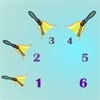When Will You Pay Me? Say the Bells of Old Bailey

Age 11 to 14 Challenge Level:

Use the interactivity to play two of the bells in a pattern. How do you know when it is your turn to ring, and how do you know which bell to ring?Constructing Triangles

Age 11 to 14 Challenge Level:

Generate three random numbers to determine the side lengths of a triangle. What triangles can you draw?Age 11 to 14 Challenge Level:

How many different symmetrical shapes can you make by shading triangles or squares?3D Stacks

Age 7 to 14 Challenge Level:

Can you find a way of representing these arrangements of balls?Auditorium Steps

Age 7 to 14 Challenge Level:

What is the shape of wrapping paper that you would need to completely wrap this model?Polygon Rings

Age 11 to 14 Challenge Level:

Join pentagons together edge to edge. Will they form a ring?Troublesome Dice

Age 11 to 14 Challenge Level:

When dice land edge-up, we usually roll again. But what if we didn't...?Icosian Game

Age 11 to 14 Challenge Level:

This problem is about investigating whether it is possible to start at one vertex of a platonic solid and visit every other vertex once only returning to the vertex you started at.Christmas Chocolates

Age 11 to 14 Challenge Level:

How could Penny, Tom and Matthew work out how many chocolates there are in different sized boxes?Dissect

Age 11 to 14 Challenge Level:

What is the minimum number of squares a 13 by 13 square can be dissected into?Tetra Square

Age 11 to 14 Challenge Level:

ABCD is a regular tetrahedron and the points P, Q, R and S are the midpoints of the edges AB, BD, CD and CA. Prove that PQRS is a square.Triangular Tantaliser

Age 11 to 14 Challenge Level:

Draw all the possible distinct triangles on a 4 x 4 dotty grid. Convince me that you have all possible triangles.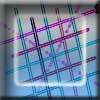Tic Tac Toe

Age 11 to 14 Challenge Level:

In the game of Noughts and Crosses there are 8 distinct winning lines. How many distinct winning lines are there in a game played on a 3 by 3 by 3 board, with 27 cells?All in the Mind

Age 11 to 14 Challenge Level:

Imagine you are suspending a cube from one vertex and allowing it to hang freely. What shape does the surface of the water make around the cube?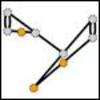Sprouts

Age 7 to 18 Challenge Level:

A game for 2 people. Take turns joining two dots, until your opponent is unable to move.Square Coordinates

Age 11 to 14 Challenge Level:

A tilted square is a square with no horizontal sides. Can you devise a general instruction for the construction of a square when you are given just one of its sides?Counting Triangles

Age 11 to 14 Challenge Level:

Triangles are formed by joining the vertices of a skeletal cube. How many different types of triangle are there? How many triangles altogether?